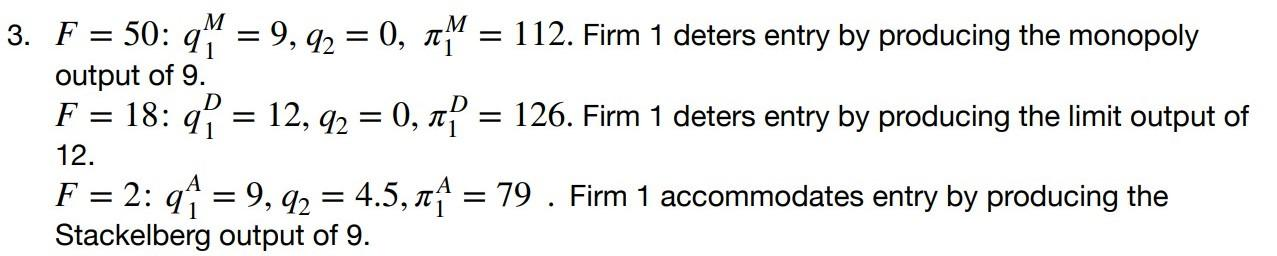### Create an Account

Home / Questions / Suppose demand is given by P=56-2Q . There are two firms with identical costs given by C(q...

# Suppose demand is given by P=56-2Q . There are two firms with identical costs given by C(qi)=20qi+F. Find the Subgame Perfect Nash equilibrium for the game with the following timing: in the first

Suppose demand is given by P=56-2Q . There are two firms with identical costs given by C(qi)=20qi+F.

Find the Subgame Perfect Nash equilibrium for the game with the following timing: in the first stage Firm 1 can commit to its output; in the second stage Firm 2 can enter and can choose its output. Do this for fixed costs equal to 50,18, and 2.Subscribe To Get Solution